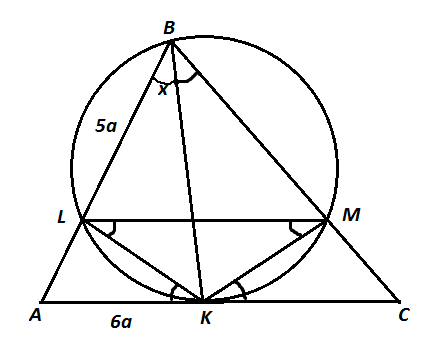# Tangent bisectors

Geometry Level 5In a triangle $ABC$, $BK$ is an angle bisector. A circle with radius $\frac{5}{3}$ passes through the vertex $B$, intersects $AB$ at a point $L,$ and is tangent to $AC$ at $K$. It is known that the length of $AC$ is $3\sqrt{3},$ and the ratio of the lengths $|AK|$ to $|BL|$ is $6:5$. The area of the triangle $ABC$ can be written as $\frac{a\sqrt{b}}{c}$, where $a$ and $c$ are coprime positive integers, and $b$ is not divisible by the square of any prime. What is the value of $a+b+c$?

×